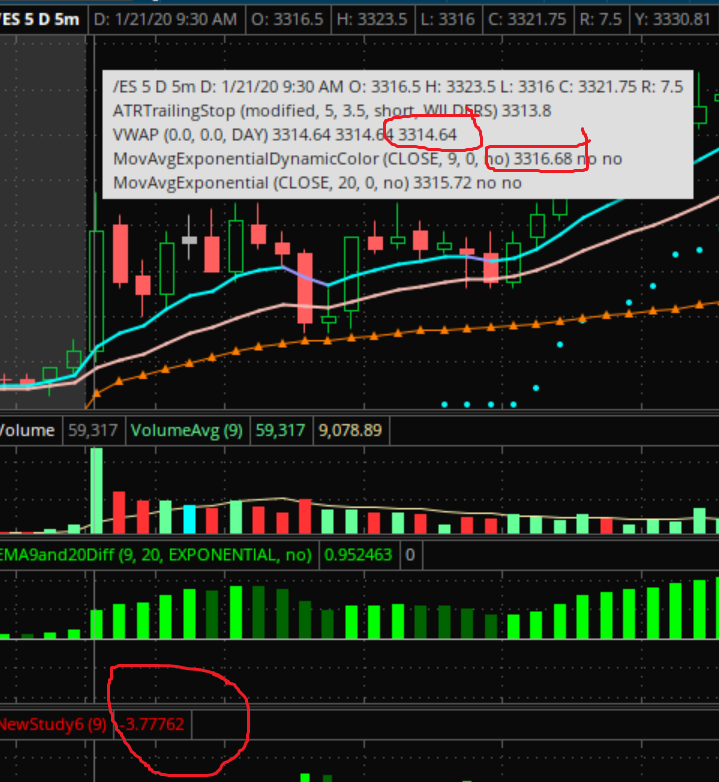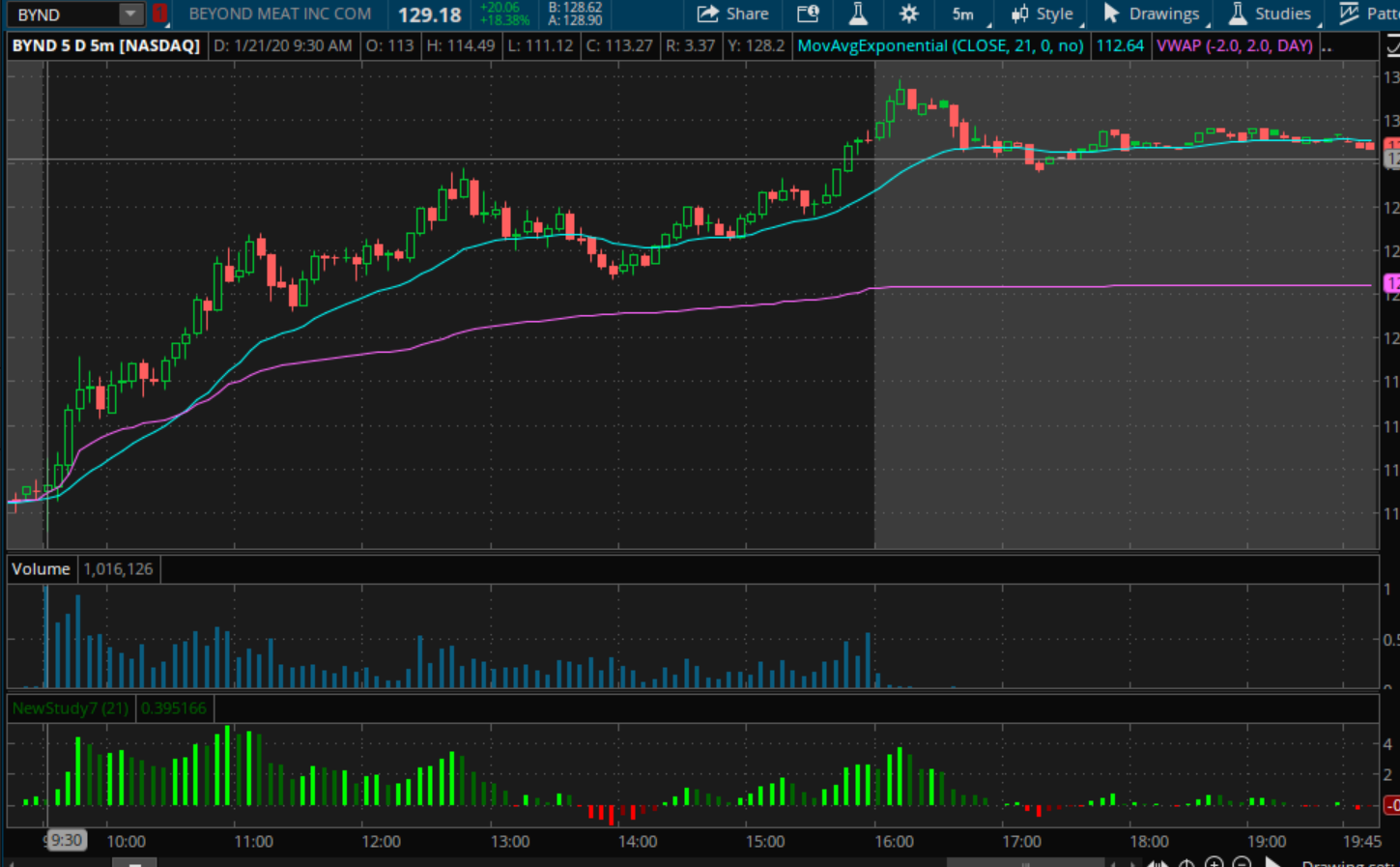# Histogram of difference between VWAP and EMA?

#### oferwo

##### New member
Hello,

I'm trying to create a histogram for the difference between the VWAP & X EMA, with 4 different colors (dark & light green , dark & light red) for positive rising, positive declining, negative declining and negative rising, but to no avail.

I've tried to copy the script from a similar histogram script I created for the difference between 2 EMA's but I can't seem to make it.

Here's the script for the difference between 2 EMA's I created.

If anyone could help me out with this, it would be GREATLY appreciated!Thanks,

Wolf
Code:
``````declare lower;

input fastLength = 12;
input slowLength = 26;
input averageType = AverageType.EXPONENTIAL;
input showBreakoutSignals = no;

plot Value = MovingAverage(averageType, close, fastLength) - MovingAverage(averageType, close, slowLength);

plot Diff = Value;
plot ZeroLine = 0;

Diff.SetPaintingStrategy(PaintingStrategy.HISTOGRAM);
Diff.SetLineWeight(3);
Diff.DefineColor("Positive and Up", Color.GREEN);
Diff.DefineColor("Positive and Down", Color.DARK_GREEN);
Diff.DefineColor("Negative and Down", Color.RED);
Diff.DefineColor("Negative and Up", Color.DARK_RED);
Diff.AssignValueColor(if Diff >= 0 then if Diff > Diff then Diff.color("Positive and Up") else Diff.color("Positive and Down") else if Diff < Diff then Diff.color("Negative and Down") else Diff.color("Negative and Up"));``````

@oferwo Here's the code that does it for you. I've used a 21 EMA. Have fun!

Code:
``````declare lower;

input length = 21;

def ema = ExpAverage(close, length);
def vwap = vwap();

plot Diff = vwap - ema;
Diff.SetPaintingStrategy(PaintingStrategy.HISTOGRAM);
Diff.DefineColor("Positive and Up", Color.GREEN);
Diff.DefineColor("Positive and Down", Color.DARK_GREEN);
Diff.DefineColor("Negative and Down", Color.RED);
Diff.DefineColor("Negative and Up", Color.DARK_RED);
Diff.AssignValueColor(if Diff >= 0 then if Diff > Diff then Diff.color("Positive and Up") else Diff.color("Positive and Down") else if Diff < Diff then Diff.color("Negative and Down") else Diff.color("Negative and Up"));``````

•BenTen
Thank you so much Tom, but for some reason it still gives me wrong values.

I tried it with a 9 EMA and the VWAP and I'm getting different values on the histogram.

For example today's 5-MIN /ES Bar at 9:30 after the close of the bar.

THE VWAP is 3314.64, the 9 EMA is 3316.68. The histogram gives the value of 3.77 instead of -2.04.

Not sure how to correct that.

That is probably due to an interpretation issue from your earlier post. Just flip the calculations and it should be what you seek. Here's the revised calculation

Code:
``plot Diff = ema - vwap;``

That just gives me -3.77 instead of 3.77 so still the wrong difference between the 9 EMA and the VWAP. I could only assume it has something to do with how the calculation of the VWAP is done by default, although the VWAP should remain consistent.Edit: The histogram with that script oscillates between green to red and back even when the relationship between the 9 EMA and the VWAP remains stable so something in the calculation is still off... ugh.. Good first attempt, though!

Last edited:
Gosh, it's all in the formula. A negative difference means is that the EMA is below the VWAP. If that really bothers you so much, just use AbsValue() and you'll get what you need.

Gosh, it's all in the formula. A negative difference means is that the EMA is below the VWAP. If that really bothers you so much, just use AbsValue() and you'll get what you need.

Tom, thanks for your help, but there seems to be a misunderstanding here. It doesn't bother me at all. I'm just getting the wrong values with that formula! 3316.68 - 3314.64 = 2.04 yet the formula as presented gives 3.77 (minus or plus doesn't matter). The formula calculates the difference wrong!

Here is another clearer image to show it with your forumla:See the relationship between the blue 21 EMA and purple VWAP?

Now look at the histogram. You can see it is not calculated well.

In any case, I appreaciate the help, but something is still off...

I have a vwap crossover script that will plot vertical lines
Code:
``````input length = 9;    #hint length: Moving Average period length.
input type = AverageType.EXPONENTIAL; #hint type: Moving Average type.

## rth is true if in regular trading hours else false.
def rth = RegularTradingStart(GetYYYYMMDD()) - GetTime() < 0 and RegularTradingEnd(GetYYYYMMDD()) - GetTime() > 0;

def volumeSum = if rth then CompoundValue(1, volumeSum + volume, volume) else 0;

def volumeVwapSum = if rth then CompoundValue(1, volumeVwapSum + volume * vwap, volume * vwap) else 0;

def volumeVwap2Sum = if rth then CompoundValue(1, volumeVwap2Sum + volume * Sqr(vwap), volume * Sqr(vwap)) else 0;

def price = if volumeSum != 0 then volumeVwapSum / volumeSum else Double.NaN;

plot VWAP = if !isNaN(close) then price else if !isNaN(close) then price else Double.NaN;

plot Avg = MovingAverage(type, if rth then close else Double.NaN, length);

VWAP.setDefaultColor(Color.PLUM);
VWAP.HideBubble();
VWAP.HideTitle();
VWAP.SetLineWeight(2);

Avg.setDefaultColor(CreateColor(0, 192, 255));
Avg.HideBubble();
Avg.HideTitle();
Avg.SetLineWeight(1);

def entry = close;

AddVerticalLine(Avg crosses above VWAP, "", Color.GREEN, 3);
AddVerticalLine(Avg crosses below VWAP, "", Color.RED, 3);``````

Last edited by a moderator:
I've had issues with the vwap() function not working consistently when used as a value in studies. I don't quite know why, but I see a lot of similar anomaly when I try to do math with the vwap(). I found a mobius script for vwap on mobile that works well, though the values it uses are subtly different than the ToS internal vwap().

When I use Mobius' code to get a value for vwap, math against vwap values works more like you'd expect it to...

Code:
``````declare lower;

input emalength = 21;

def ema = ExpAverage(close, emalength);

# Intraday VWAP for Mobile or PC
# Mobius
# V01.08.2017
# Revision of VWAP using the new getTime()function for Mobile or PC.
def RTH = GetTime() >= RegularTradingStart(getYYYYMMDD());
def VolData = if RTH and !RTH
then vwap
else if RTH
then CompoundValue(1, VolData + vwap,
vwap)
else VolData;
def length = if RTH and !RTH
then 1
else if RTH
then CompoundValue(1, length + 1, 1)
else length;
# CHANGE plot to def in the following line for this study
def vwap = if RTH
then VolData / length
else Double.NaN;
# END Mobius Code

plot Diff = ema - vwap;
Diff.SetPaintingStrategy(PaintingStrategy.HISTOGRAM);
Diff.DefineColor("Positive and Up", Color.GREEN);
Diff.DefineColor("Positive and Down", Color.DARK_GREEN);
Diff.DefineColor("Negative and Down", Color.RED);
Diff.DefineColor("Negative and Up", Color.DARK_RED);
Diff.AssignValueColor(if Diff >= 0 then if Diff > Diff then Diff.color("Positive and Up") else Diff.color("Positive and Down") else if Diff < Diff then Diff.color("Negative and Down") else Diff.color("Negative and Up"));``````

I get a histogram that looks decidedly more like what you'd expect.

Last edited:
•oferwo
thats great I also came across a vwap close script and loaded it
Previous Days VWAP Close
Code:
``````def x = barNumber();
def c = close;
def nan = double.nan;
def vwap = reference VWAP();
def Pc = if getTime() crosses above RegularTradingEnd(getYYYYMMDD())
then Round(VWAP / tickSize(), 0) * tickSize()
else Pc;
def Pcx = if getTime() crosses above RegularTradingEnd(getYYYYMMDD())
then x
else Pcx;
plot Pc_ = if x >= highestAll(Pcx)
then highestAll(if isNaN(c[-1])
then Pc
else nan)
else nan;
Pc_.SetStyle(Curve.Long_Dash);
Pc_.SetDefaultColor(Color.White);
Pc_.HideBubble();
Pc_.HideTitle();
AddChartBubble(x == highestAll(x), Pc, "PC", Pc_.TakeValueColor(), 1);``````

Last edited by a moderator:
•oferwo
Thank you, guys! That's very helpful!

hi!
how do i write a script on my scan that include a a dollar difference or more between 9EMA and VWAP?

like the value of 9EMA is \$10
and the VWAP is \$9
then it will show up on my scan those stocks that have a difference of more than \$1 or more

### Not the exact question you're looking for?

87k+ Posts
263 Online## The Market Trading Game Changer

Join 2,500+ subscribers inside the useThinkScript VIP Membership Club
• Exclusive indicators
• Proven strategies & setups
• Private Discord community
• Exclusive members-only content
• 1 full year of unlimited support

What is useThinkScript?

useThinkScript is the #1 community of stock market investors using indicators and other tools to power their trading strategies. Traders of all skill levels use our forums to learn about scripting and indicators, help each other, and discover new ways to gain an edge in the markets.

How do I get started?

We get it. Our forum can be intimidating, if not overwhelming. With thousands of topics, tens of thousands of posts, our community has created an incredibly deep knowledge base for stock traders. No one can ever exhaust every resource provided on our site.

If you are new, or just looking for guidance, here are some helpful links to get you started.

What are the benefits of VIP Membership?